# Intersection of a Straight Line with a Plane

### Intersection of a straight line with a plane in general position

Let us recall how we determine the position of a point T that lies in the plane P- the point must lie on the straight line that belongs to this plane. The construction of the intersection point P of a straight line p with a plane P (assumed that the line doesn't belong to that plane or is parallel to it and the plane is in general position) is based on finding the line of the plane P that intersects the line p in the point of intersection of p and P .

To construct the point of intersection P of a straight line and a plane in general position we proceed as follows:

• draw an auxiliary plane Σ  through the given line p;
• construct the line of intersection f of the planes P and Σ .
• determine the position of the point P of intersection of the given line p and the constructed line f .

Animation below shows this procedure.
Click on the image to start the animation.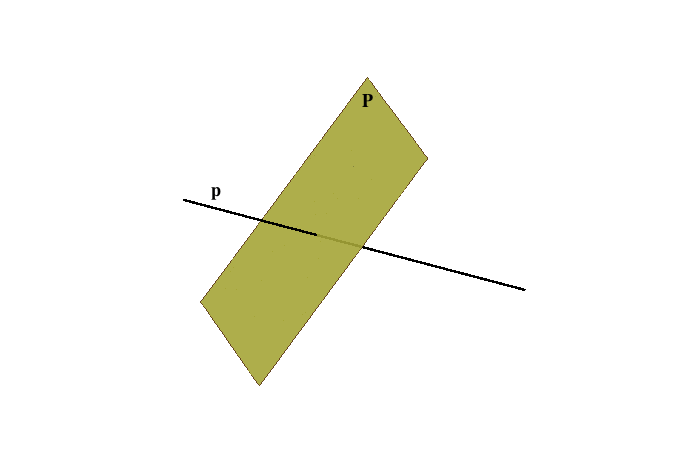1.Σ , p ⊂ Σ 2.f = P ∩ Σ 3.P = p ∩ f = p ∩ P
How this procedure is done in Monge's method:1. Intersection of a straight line and a plane using a plane in general position as auxiliary plane.2. Intersection of a straight line and a plane using a projecting plane as auxiliary plane.

The comparison of the two given constructions are presented in the figures below. Because of the simplicity of the second approach we will always use projecting planes as auxiliary planes in the construction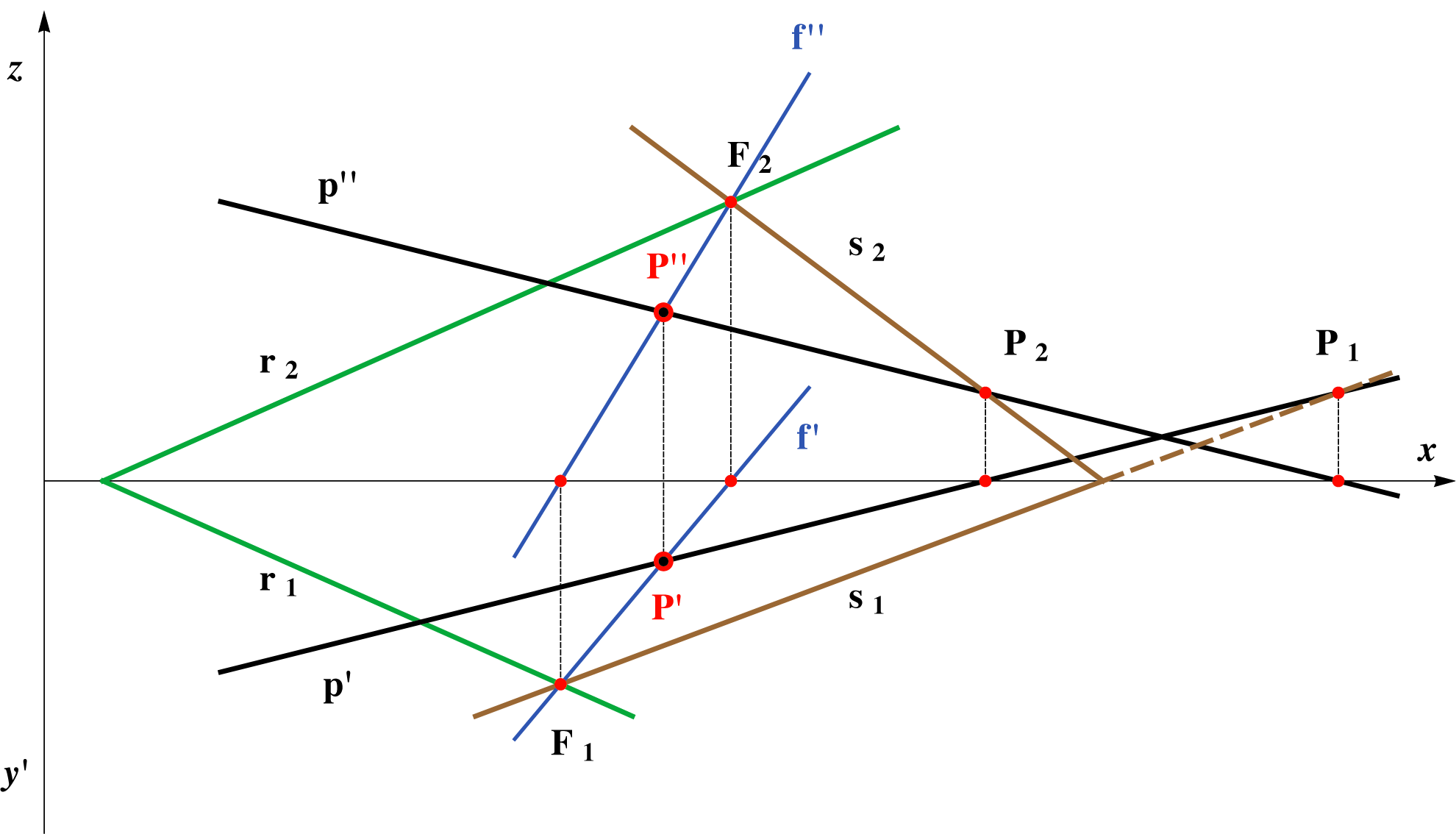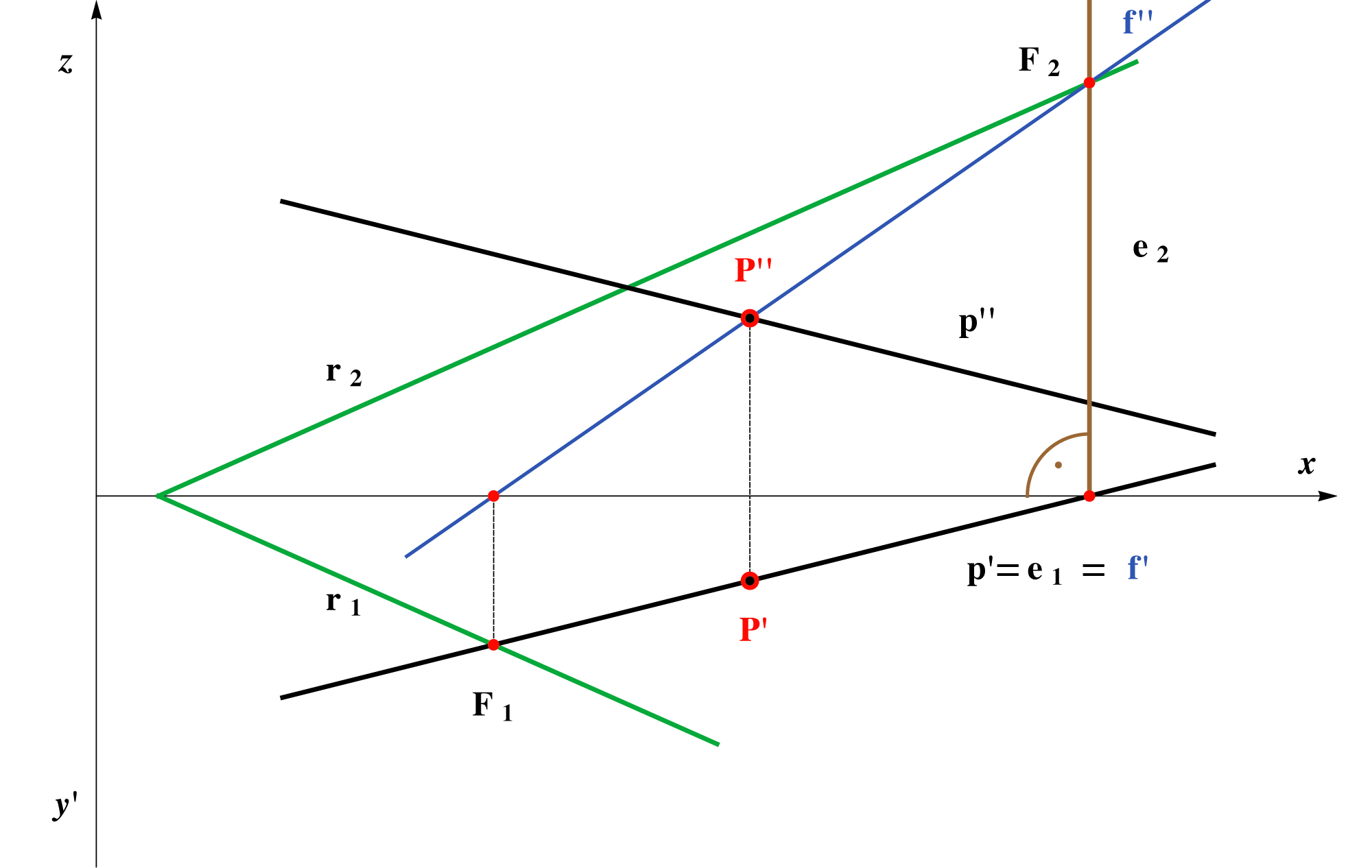Auxiliary plane Σ is an oblique plane. Auxiliary plane E is a projecting plane.

Assignment 1: Construct the projections of the point of intersection of the line  p=AB[A(0,4,3);B(6,–1,–1)] with the plane P(,3,2).As an axiliary plane use the vertical projecting plane E.Denote the visibility of p with regard to the plane P in both projections.

Assignment 2: Construct the projections of the point of intersection of the line a=A1A2[A1(5,3,0);A2(–1,0,4)]  Δ(3,2,–3).As an axiliary plane use the horizontal projecting plane E.Denote the visibility of p with regard to the plane Δ in both projections.

### The intersection of a straight line with a projecting plane

When we are dealing with projecting planes, we must remember that they are perpendicular to one (or more) planes of projection, and in that projection they project onto a line (corresponding trace). Therefore, that projection of the intersection of a line and that plane also lies on that trace. See the images below.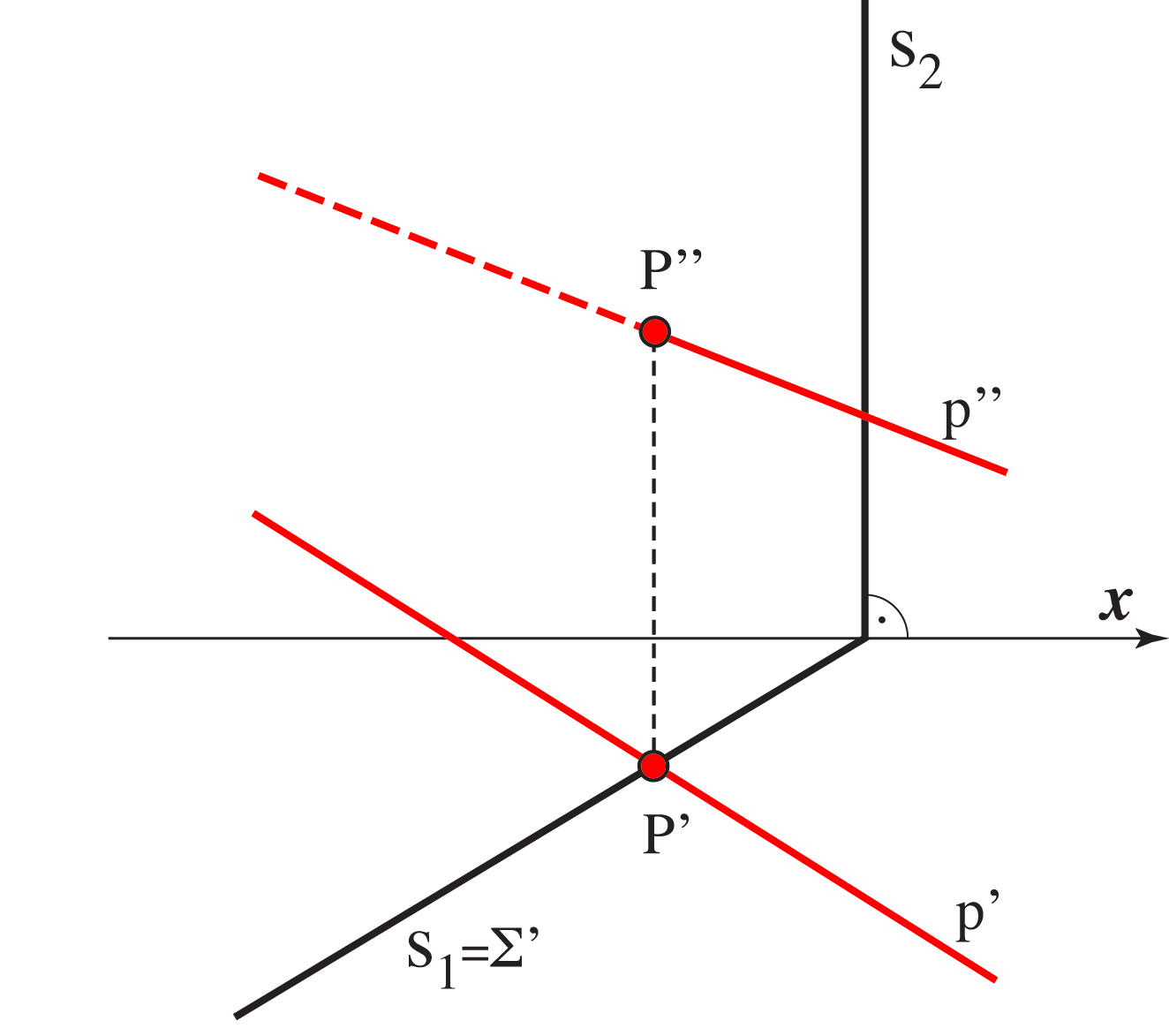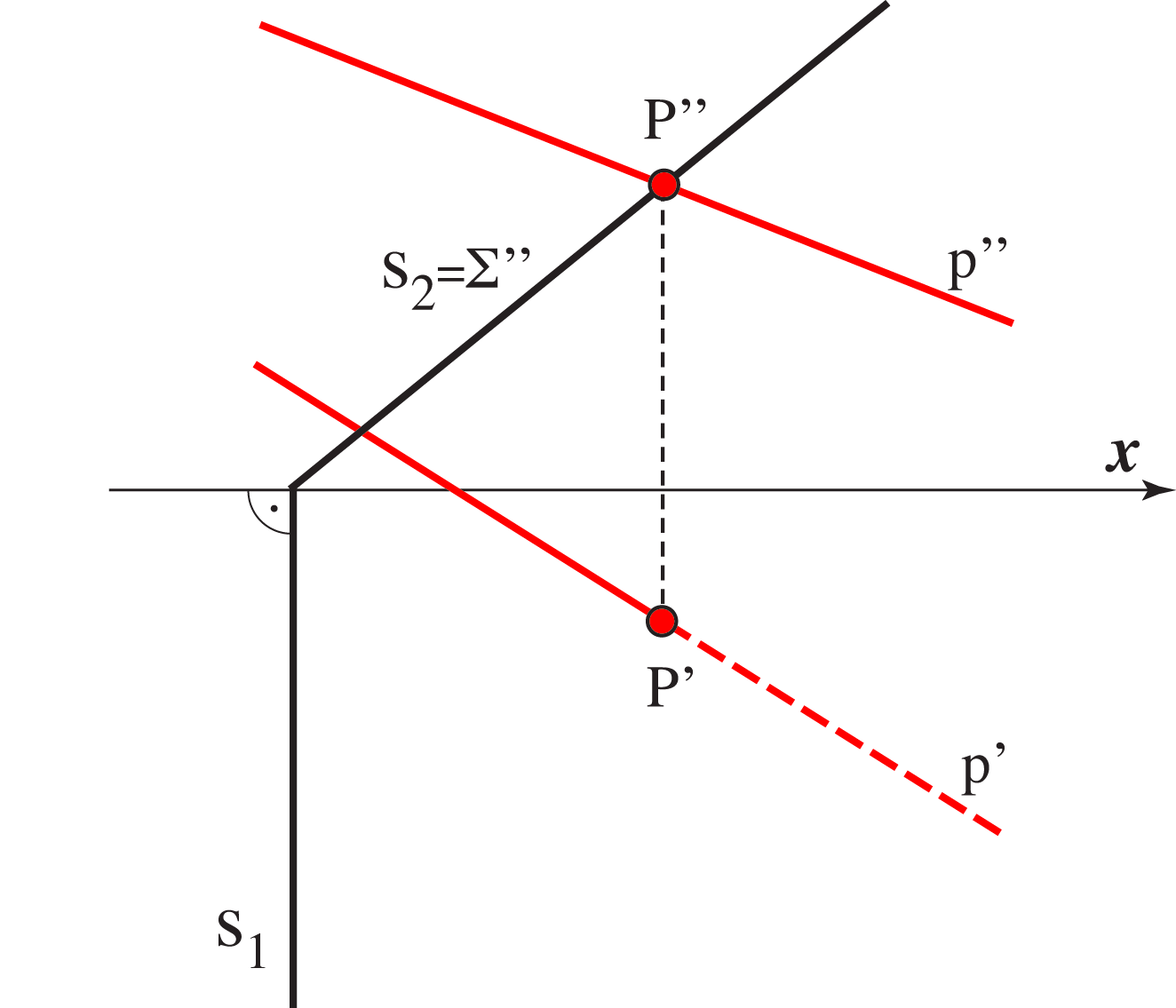Construction of the intersection of the line p with horizontal projecting plane Σ. Construction of the intersection of the line p with vertical projecting plane Σ.

Created by Sonja Gorjanc, translated by Helena Halas and Iva Kodrnja - 3DGeomTeh - Developing project of the University of Zagreb